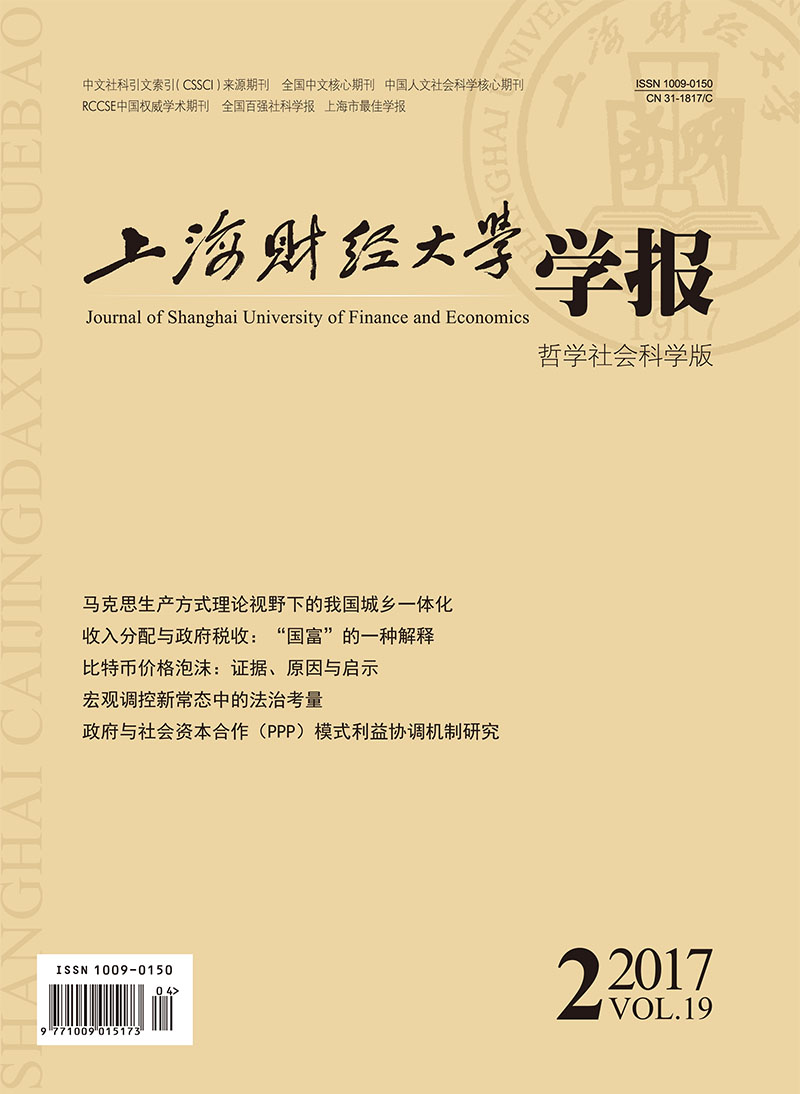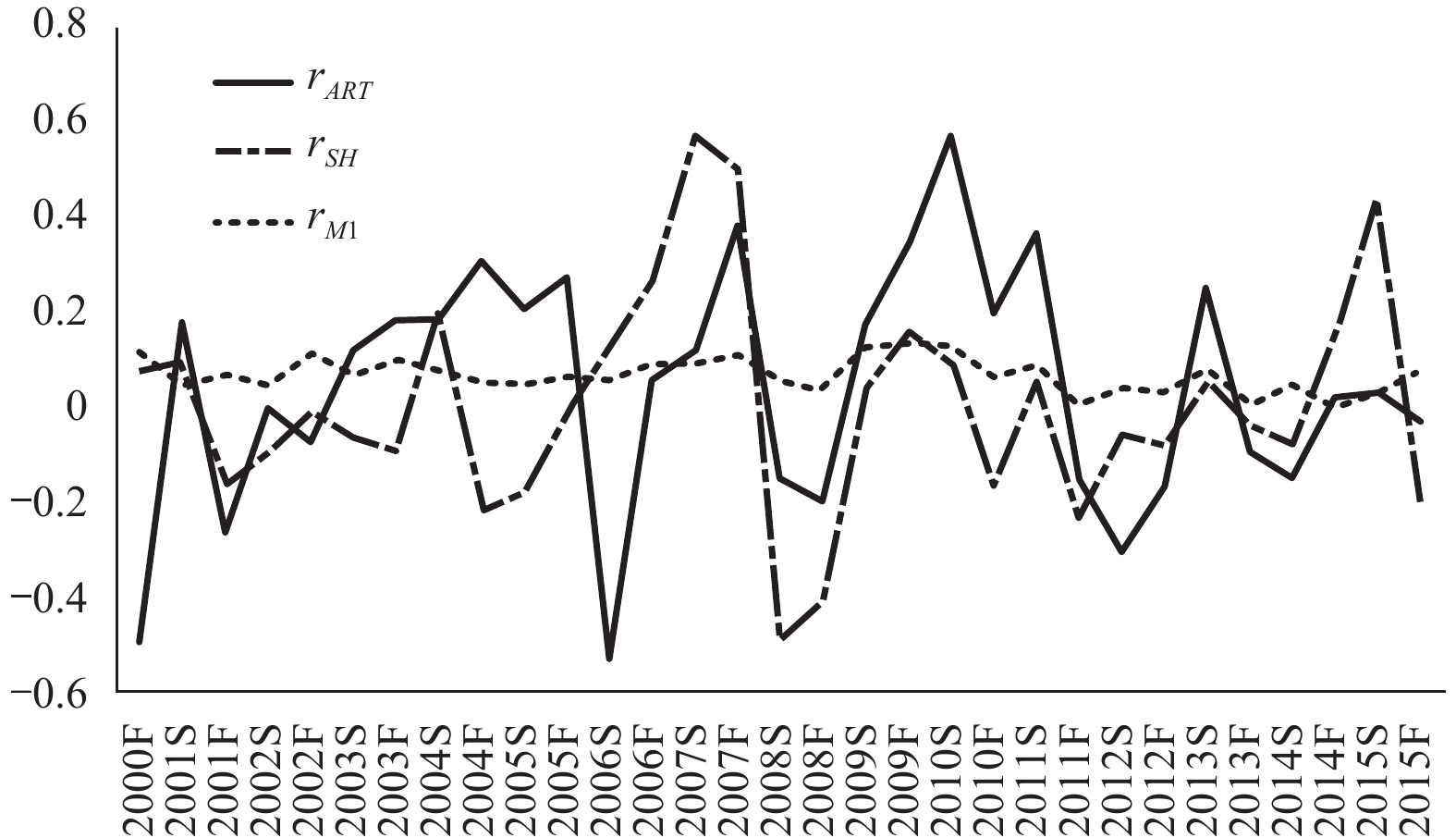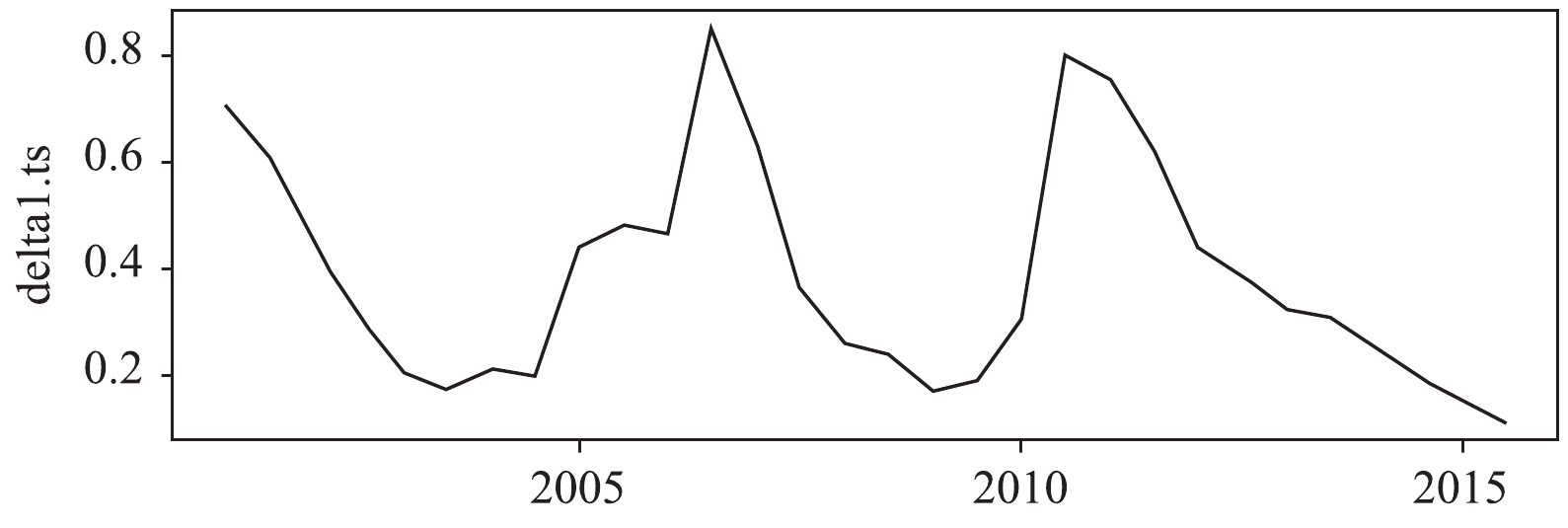﻿ 中国艺术品市场投资真的是高收益、低风险吗？
 上海财经大学学报2017, Vol. 19Issue (2): 63-760

#### 文章信息上海财经大学2017年19卷第2期

Qiao Mingzhe, Fang Yan, Huang Xiangyun, Zhang Weiqian

Does China’s Art Market Investment Really Have High Returns and Low Risks?

Journal of Shanghai University of Finance and Economics, 2017, 19(2): 63-76.

### 文章历史《上海财经大学学报》
2017第19卷第2期

1.上海对外经贸大学 金融管理学院,上海 201620;
2.南开大学 商学院,天津 300071;
3.上海财经大学 统计与管理学院,上海 200433

（一）艺术品市场投资收益及其与证券市场的比较

①数据来源:《TEFAF Art Market Report 2015》,TEFAF Maastricht,2015.

（二）艺术品市场投资风险及其与证券市场的比较

“高收益,高风险”是投资活动的黄金法则.艺术品投资不同于一般投资品,要求投资者具备丰富的专业知识和鉴别能力,投资门槛较高.相对于证券、债券等金融投资而言,艺术品的流动性和变现能力较差,更容易受到经济萧条等不利因素的影响,而产生更高的风险.

Worthington和Higgs（2003）、Atukeren和Seçkin（2009）也分别比较了艺术品市场和全球股票市场收益率的方差,他们都发现艺术品市场收益的方差高于股票市场,表现出更高的投资风险.Mandel（2009）的研究表明,艺术品收益率的方差是道琼斯工业股票指数和企业债券的2到3倍,即艺术品投资收益的风险比股票和债券高很多.Hodgson和Seçkin（2012）针对加拿大市场的实证分析也显示,艺术品资产收益率的标准差为13.29%,相对于传统资本市场而言风险程度更高.

（三）艺术品市场投资收益与证券市场投资收益的相关性

（一）变量选取和数据说明

 ${r_t} = {\rm log}\left( {{P_t}} \right) - {\rm log}\left( {{P_{t - 1}}} \right) - CP{I_t}$ (1)

①互联网地址:www.artron.net.

 变量 P值 结论 rART 0.01* 平稳 rSH 0.016* 平稳 rM1 0.01* 平稳 注:*代表在5%水平上平稳.

（二）艺术品市场和上证综指的收益率及其风险图 1 艺术品指数收益、上证综指收益与M1增长率的时序图 注:横轴中“F”和“S”分别表示“秋季”和“春季”,例如“2000F”表示2000年秋季.

 变量 均值（%） 中位数（%） 最大值（%） 最小值（%） 标准差（%） 标准离差率（%） 偏度 超额峰度 相关系数矩阵 1 2 3 rART 4.35 5.96 57.38 –53.37 25.74 5.92 –0.307 –0.415 1 rSH 0.52 –1.79 56.53 –49.37 23.50 45.19 0.415 0.257 0.278 1 rM1 6.74 6.36 13.33 –0.14 3.68 0.55 0.028 –0.873 0.392 0.338 1 注:N=31,2000年秋至2015年秋（以2000年春为基准年份）.

 $\begin{array}{l}{r_{ART,t}} = {\varphi _{ART,0}} + {\varphi _{ART,1}}{r_{ART,t - 1}} + {a_{ART,t}}\\{a_{ART,t}} = {\sigma _{ART,t}}{\varepsilon _{ART,t}}\\\sigma _{ART,t}^2 = {\alpha _{ART,0}} + {\alpha _{ART,1}}\sigma _{ART,t - 1}^2 + {\beta _{ART,t}}\varepsilon _{ART,t - 1}^2\end{array}$ (2)
 $\begin{array}{l}{r_{SH,t}} = {\varphi _{SH,0}} + {\varphi _{SH,1}}{r_{SH,t - 1}} + {\varphi _{SH,2}}{r_{SH,t - 2}} + {a_{SH,t}}\\{a_{SH,t}} = {\sigma _{SH,t}}{\varepsilon _{SH,t}}\\\sigma _{SH,t}^2 = {\alpha _{SH,0}} + {\alpha _{SH,1}}\sigma _{SH,t - 1}^2 + {\beta _{SH,t}}\varepsilon _{SH,t - 1}^2\end{array}$ (3)

 检验方法 均值 标准差 t检验 Wilcoxon rank sum Welcht检验 备择假设 rART ≠rSH rART >rSH rART >rSH rART >rSH p值 0.542 0.272 0.16 0.038

（三）艺术品市场和上证综指收益率的动态相关性

 变量 F值 p-value 变量 F值 p-value 变量 F值 p-value rART →rSH 2.547 0.122 rSH →rART 0.314 0.579 rM1 →rART 0.563 0.026 rART →rM1 0.083 0.775 rSH →rM1 0.067 0.798 rM1 →rSH 0.225 0.639

 $\left[ {\begin{array}{*{20}{c}}{{r_{ART,t}}}\\{{r_{SH,t}}}\\{{r_{M1,t}}}\end{array}} \right] = {\Phi _0} + {\Phi _1} \times \left[ {\begin{array}{*{20}{c}}{{r_{ART,t - 1}}}\\{{r_{SH,t - 1}}}\\{{r_{M1,t - 1}}}\end{array}} \right] + {\varepsilon _t}$ (4)
 ${L^{ - 1}} \times \left[ {\begin{array}{*{20}{c}}{{r_{ART,t}}}\\{{r_{SH,t}}}\\{{r_{M1,t}}}\end{array}} \right] = {L^{ - 1}}{\Phi _0} + {L^{ - 1}}{\Phi _1} \times \left[ {\begin{array}{*{20}{c}}{{r_{ART,t - 1}}}\\{{r_{SH,t - 1}}}\\{{r_{M1,t - 1}}}\end{array}} \right] + {L^{ - 1}}{\varepsilon _t}$ (5)

${L^{ - 1}} = \left[ {\begin{array}{*{20}{c}}1&0&0\\0&1&{{ {1.05}}{{\left( { {0.23}} \right)}^*}}\\0&{ - {{{ 0.9}}}{{\left( { {0.21}} \right)}^*}}&1\end{array}} \right]$ , ${\Phi _0} = \left[ {\begin{array}{*{20}{c}}{ - 12.95\left( {9.32} \right)}\\{0.87\left( {9.52} \right)}\\{{ {4.94}}{{\left( { {1.51}} \right)}^*}}\end{array}} \right]$ ,

${\Phi _1} = \left[ {\begin{array}{*{20}{c}}{ - 0.039\left( {0.18} \right)}&{0.0002\left( {0.19} \right)}&{{ {2.88}}{{\left( { {1.29}} \right)}^*}}\\{ - 0.27\left( {0.18} \right)}&{0.3\left( {0.20} \right)}&{0.045\left( {1.31} \right)}\\{ - 0.0096\left( {0.03} \right)}&{0.0096\left( {0.03} \right)}&{0.249\left( {0.207} \right)}\end{array}} \right]$

 $\begin{array}{l}{r_{ART,t}} = - 12.95 - 0.039 \times {r_{ART,t - 1}} + 0.002 \times {r_{SH,t - 1}} + {2.88^*} \times {r_{M1,t - 1}}\\\left( {9.32} \right)\left( {0.18} \right)\left( {0.20} \right)\left( {1.29} \right)\end{array}$ (6)
 $\begin{array}{l}{r_{SH,t}} = 6.07 - {1.05^*} \times {r_{M1,t}} - 0.28 \times {r_{ART,t - 1}} + 0.31 \times {r_{SH,t - 1}} + 0.307 \times {r_{M1,t - 1}}\\\left( {9.73} \right)\left( {0.23} \right)\left( {0.19} \right)\left( {0.20} \right)\left( {1.33} \right)\end{array}$ (7)
 $\begin{array}{l}{r_{M1,t}} = 4.16 + {0.9^*} \times {r_{SH,t}} + 0.24 \times {r_{ART,t - 1}} - 0.26 \times {r_{SH,t - 1}} + 2.08 \times {r_{M1,t - 1}}\\\left( {8.93} \right)\left( {0.21} \right)\left( {0.18} \right)\left( {0.20} \right)\left( {1.23} \right)\end{array}$ (8)图 2 脉冲响应分析结果

（四）艺术品市场和上证综指的风险对冲分析

 ${r_{ART,t}} - {r_{f,t}} = \alpha + \beta \times \left( {{r_{SH,t}} - {r_{f,t}}} \right) + {\varepsilon _t}$ (9)

 $\delta _t^* = {h_{ARTSH,t}}/{h_{ART,t}}$ (10)

 ${r_{Hedged,t}} = {r_{SH,t}} - \delta _t^*{r_{ART,t}}$ (11)图 3 ART与SH的动态最优套期保值比率

 $HE = \frac{{Va{r_{unhedged}} - Va{r_{hedged}}}}{{Va{r_{unhedged}}}}$ (12)

（1）国内艺术品市场的投资收益率高于证券市场,但不显著.2000–2015年间雅昌艺术品指数的平均收益率为4.35%,约为同期上证指数平均收益率的8倍,但是分组比较统计不显著.国内艺术品市场投资收益率高于发达国家研究（Mei和Moses,2005；Hodgson,2011）得出的收益水平,与新兴经济体波兰的艺术品市场5.51%的名义收益水平（Lucińska,2015）以及国内相关研究结论接近,为艺术品市场投资收益水平与市场成熟度相关的理论假说提供了证据.不过本文结论低于国内相关研究15.8%（石阳和李曜,2013）和10.96%（周思达等,2014）的平均收益水平.其原因可能在于:首先,2013年以来国内艺术品市场经历了大幅度调整；其次,本文使用的是低于名义收益率的实际收益率.总之,国内艺术品市场的平均收益水平大幅高于同期证券市场,两者的分组比较不显著可能是由于样本较少所致.

（2）国内艺术品市场投资的绝对风险高于证券市场,但相对风险低于证券市场.在实证分析中,我们发现艺术品市场的绝对风险（标准差25.74%）高于证券市场（标准差23.50%）2.24个百分点,与Mei和Moses（2005）等国外研究的结论一致.风险较高可能是由于艺术品的天然异质性使得其投资收益的不确定性增加所致.另外,本文发现国内艺术品市场收益率的标准差低于Mei和Moses（2005）等国外研究的水平,表明现阶段国内艺术品市场的投资风险低于发达国家.

（3）国内艺术品市场投资收益与证券市场投资收益的相关性较低,且货币增长率对两者的冲击存在不同的时滞,可以通过投资组合对冲风险.本文发现国内艺术品市场与证券市场存在弱正相关关系,该结论和国外（Chanel等,1994；Worthington和Higgs,2003；Mei和Moses,2005；Hodgson和Seçkin,2012）由CAPM模型与相关分析得出的结论基本一致.与发达国家研究中α接近于0不同,国内艺术品市场的α明显大于0,表明国内艺术品市场相对于证券市场而言更加独立,即两者组合具有更佳的风险对冲效果.套期保值比率和对冲有效性指标的分析结果表明,国内艺术品市场和证券市场具有较好的对冲效果（HE=0.742）,进一步印证了CAPM模型的结论.因此,国内投资者可以通过构建由一定比例艺术品资产和证券资产构成的投资组合,实现风险的有效对冲.

  黄隽, 唐善才. 艺术品金融市场:文献综述[J].国际金融研究,2014(2).  牟建平. 艺术品的“8大财经特征”（下）[J].艺术市场,2008(4).  石阳, 李曜. 中国艺术品投资收益——整体特征与杰作效应[J].金融研究,2013(12).  严俊. 艺术品市场的定价机制——关于美学价值与艺术声誉的理论讨论[J].上海财经大学学报,2013(4).  周思达, 贺炜, 杨胜刚. 书画艺术品市场周期与投资收益的研究[J].湖南大学学报(社会科学版),2014(2).  Atukeren E,Seçkin A. An analysis of the price dynamics between the Turkish and the international paintings markets[J].Applied Financial Economics,2009,19(21):1705–1714.  Baillie R T,Myers R J. Bivariate GARCH estimation of the optimal commodity futures hedge[J].Joumal of Applied Econometrics,1991,6(2):109–124.  Baumol W J. Unnatural value:Or art investment as floating crap game[J]. The American Economic Review,1986,76(2):10–14.  Blanchard O J,Quah D. The dynamic effects of aggregate supply and demand disturbances[J]. The American Economic Review,1989,79(4):655–673.  Buelens N,Ginsburgh V. Revisiting Baumol’s ‘Art as floating crap game’[J].European Economic Review,1993,37(7):1351–1371.  Campbell R,Pullan J.Diversification into art mutual funds[A].Gregoriou G N.Diversification and portfolio management of mutual funds[C].New York:Palgrave Macmillan,2007:1–17.  Chanel O,Gerard-Varet L A,Ginsburgh V. Prices and returns on paintings:An exercise on how to price the priceless[J].The Geneva Papers on Risk and Insurance Theory,1994,19(1):7–21.  Ederington I H. The hedging performance of the new futures markets[J].The Journal of Finance,1979,34(1):157–170.  Frey B S,Eichenberger R. On the return of art investment return analyses[J].Journal of Cultural Economics,1995,19(3):207–220.  Goetzmann W N. Accounting for taste:Art and the financial markets over three centuries[J]. American Economic Review,1993,83(5):1370–1376.  Hodgson D. An Analysis of Pricing and returns in the market for French Canadian paintings[J].Applied Economics,2011,43(1):63–73.  Hodgson D J,Seçkin A. Dynamic price dependence of Canadian and international art markets:An empirical analysis[J].Empirical Economics,2012,43(2):867–890.  Johnson L L. The theory of hedging and speculation in commodity futures[J].Review of Economic Studies,1960,27(3):139–151.  Lucińska A. The art market in the European Union[J].International Advances in Economic Research,2015,21(1):67–79.  Mandel B R. Art as an investment and conspicuous consumption good[J].American Economic Review,2009,99(4):1653–1663.  Mei J,Moses M. Art as an investment and the underperformance of masterpieces[J].American Economic Review,2002,92(5):1656–1668.  Mei J,Moses M. Beautiful asset:Art as investment[J]. Journal of Investment Consulting,2005,7(2):45–51.  Pesando J E. Art as an investment:The market for modern prints[J]. American Economic Review,1993,83(5):1075–1089.  Renneboog L,Spaenjers C. Buying beauty:On prices and returns in the art market[J].Management Science,2013,59(1):36–53.  Worthington A C,Higgs H. Art as an investment:Short and long-term comovements in major painting markets[J].Empirical Economics,2003,28(4):649–668.
Does China’s Art Market Investment Really Have High Returns and Low Risks?
Qiao Mingzhe1,2, Fang Yan1,3, Huang Xiangyun1, Zhang Weiqian1
1.School of Finance & Management,Shanghai University of International Business and Economics,Shanghai 201620,China;